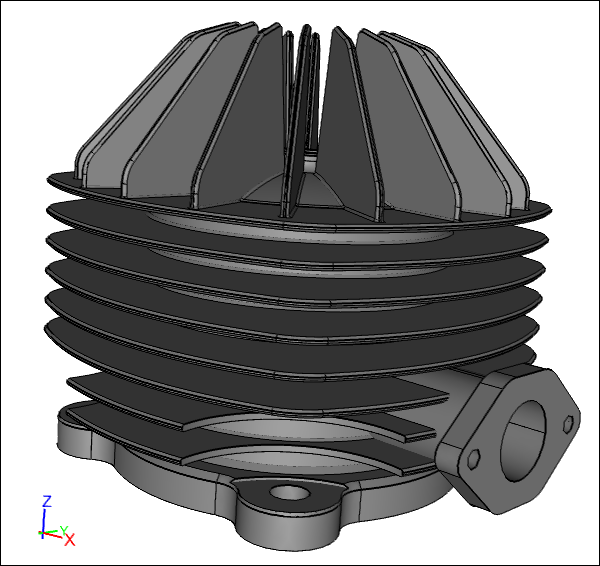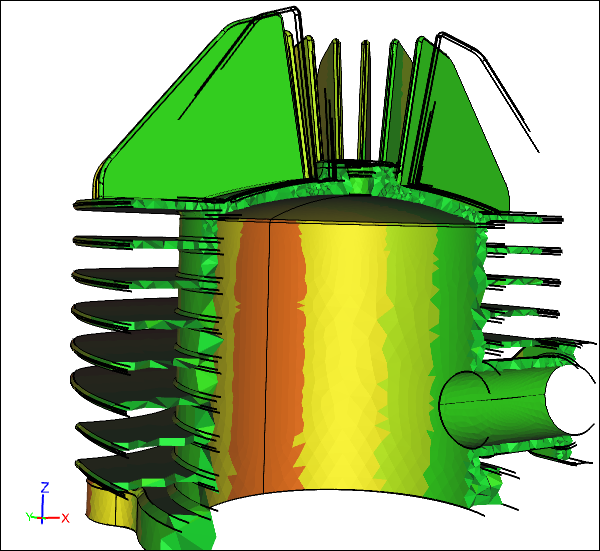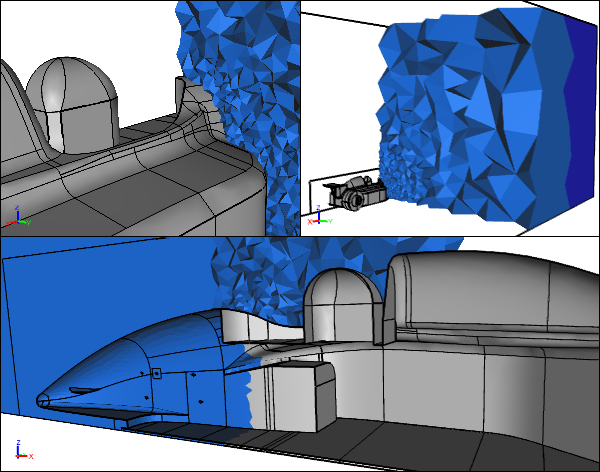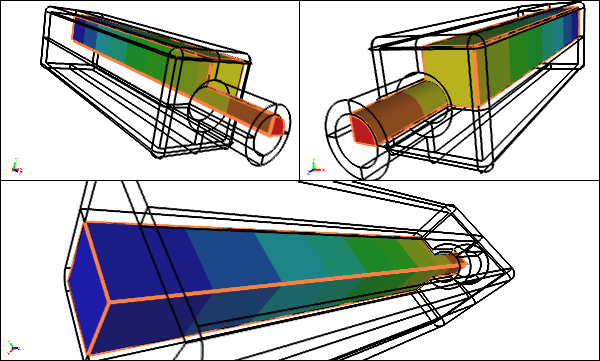# CFD Flow Volumes and Solid Models

Solid modeling is the dominant form of virtual object representation underpinning nearly all 3D Computer-Aided Design (CAD) systems. The strength of a solid model is that it defines a water tight (manifold) unambiguous object which implicitly delineates between the inside and the outside of an object simply by the alignment of the boundaries (B-rep or BREP) that define it. This makes solid modeling an ideal basis for virtual analysis techniques, e.g., stress analysis and Computational Fluid Dynamics (CFD). Also solid modeling provides a relatively straightforward path to manufacture through CNC machines and, more recently, 3D printing.Cylinder Head Solid ModelBoundary Representation (B-rep or BREP)

### Stress Analysis

A typical solid model, as used in mechanical engineering, represents a 3D region within a set of boundaries that will eventually become a solid physical object, e.g., a cylinder head.Cylinder Head Stress ModelSubstance (aluminum) modeled within the cylinder head

This form of solid model is the direct basis for most stress analysis simulations, where the substance (material) under analysis is likely a solid metal. If there is geometric symmetry then often a half solid model can be used for the analysis, reducing the computer resource requirements and improving the turnaround speed.

### Computational Fluid Dynamics

CFD is similar to stress analysis in that it requires a solid model to perform an analysis. However, the substance (e.g., air) being modeled does not lie within the object, but everywhere outside the object. For the external airflow over a car that means defining a solid model (often referred to as a flow volume or flow domain) that defines a region around the car that extends many car lengths away in all directions so as to avoid interfering with the localized airflow over the car - just as the walls in a wind tunnel are ideally positioned far from the model under test. Typically the solid model for an external CFD analysis consists of the object of interest subtracted from a large box, similar to the mold you would create to cast the object.External CFD Flow VolumeSubstance (air) modeled outside the car. Half model due to symmetry.

STEP (.stp) is usually a good choice to exchange geometry between applications. STEP maintains geometric accuracy and retains the topological connections that define a solid.

For internal flows the same rules apply. The internal water flow through a pipe requires the solid model to represent the water region within the pipe. Typically you take the inner wall of the pipe that will be in contact with the water and cap the inlet and outlet to form a solid.Internal CFD Flow VolumeSubstance (water) modeled within duct. Quarter model due to symmetry.

Geometric symmetry does not necessarily equate to fluid symmetry. A symmetrical car in a headwind is a candidate for a symmetry half model, but a car in a cross wind requires the full model as the flow will not be symmetric relative to the symmetry plane of the car. Given the relatively large simulation requirements for external CFD simulations, symmetry is a significant resource saver if applicable.